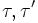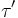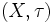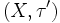# Category:Coarsening-preserved properties of topological spaces

This category lists coarsening-preserved properties of topological spaces. This means that if$X$ has topologies$\tau, \tau'$ and$\tau'$ is a coarser topology than$\tau$, then:$(X,\tau)$ has the property implies that$(X,\tau')$ has the property